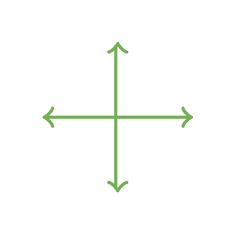100

6 x 8=

48

100What is this angle called?

Acute

100

1/4 + 2/4=

3/4

100

10 x 10=

100

100

What mixed number is equal to 14/4?

3 1/2  or 3 2/4

200

7 x 9=

63

200

A right angle is at what degrees?

90 degrees

200

4/8 + 13/8 =?

17/8 or 2 1/8

200

200 x 2 =

400

200

1/6 + 4/6 = ?

5/6

300

8 x 8

64

300

What is the angle called that is more than 90 degrees?

obtuse

300

5/7 - 4/7 = ?

1/7

300

120 + 120 + 20 + 20 + 1=

281

300

2,481 + 3,394=

5,875

400

16 x 8 =

128

400What do you call this image?

perpendicular lines

400

Stella ate 1 1/2 oranges before lunch and ate another 2 1/2 oranges afterward. How many oranges did she eat?

4 oranges

400

40 times 10

400

400

What is 56,238 rounded to the nearest thousand?

56,000

500

25 x 11=

275

500

What kind of lines are these?Parallel

500

3 X 3/8=

9/8 or 1 1/8

500

1001- 901=

100

500

20 dogs went for a walk.  They walked 260 miles in all. How far did each dog walk?

13 miles

Click to zoom